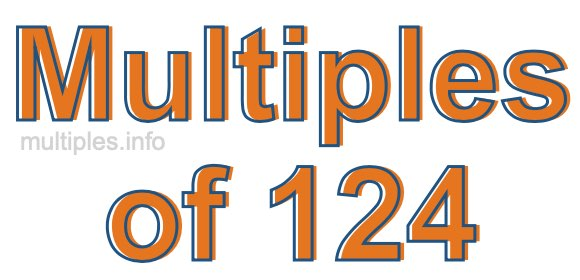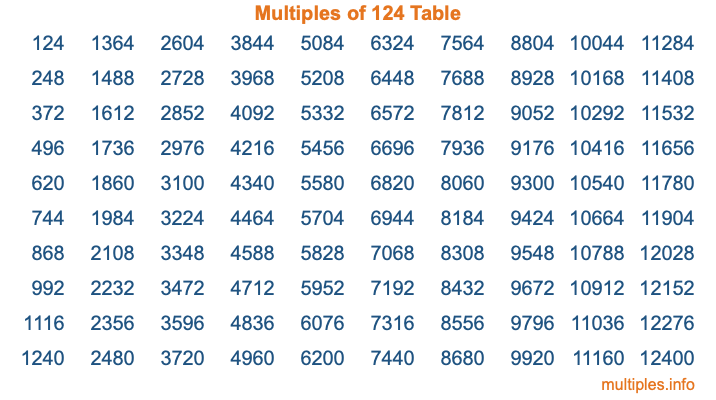Multiples of 124Welcome to the Multiples of 124 page. Here we will first teach you everything you will ever need to know about the multiples of 124, and then give you a study guide summary of everything we taught you to make sure you remember it all. Use this page to look up facts and learn information about the multiples of 124. This page will make you a multiples of one hundred twenty-four expert!

Definition of Multiples of 124
Multiples of 124 are all the numbers that when divided by 124 equal an integer. Each of the multiples of 124 are called a multiple. A multiple of 124 is created by multiplying 124 by an integer.

Therefore, to create a list of multiples of 124, you start with 1 multiplied by 124, then 2 multiplied by 124, then 3 multiplied by 124, and so on for as long as you want. Thus, the list of the first five multiples of 124 is 124, 248, 372, 496, and 620. To see a larger list of multiples of 124, see the printable image of Multiples of 124 further down on this page. We also have a category where you can choose any nth multiple of 124.

Multiples of 124 Checker
The Multiples of 124 Checker below checks to see if any number of your choice is a multiple of 124. In other words, it checks to see if there is any number (integer) that when multiplied by 124 will equal your number. To do that, we divide your number by 124. If the the quotient is an integer, then your number is a multiple of 124.

Is  a multiple of 124?

Least Common Multiple of 124 and ...
A Least Common Multiple (LCM) is the lowest multiple that two or more numbers have in common. This is also called the smallest common multiple or lowest common multiple and is useful to know when you are adding our subtracting fractions. Enter one or more numbers below (124 is already entered) to find the LCM.

Check out our LCM Calculator if you need more details about the Least Common Multiple or if you need the LCM for different numbers for adding and subtraction fractions.

nth Multiple of 124
As we stated above, 124 is the first multiple of 124, 248 is the second multiple of 124, 372 is the third multiple of 124, and so on. Enter a number below to find the nth multiple of 124.

th multiple of 124

Multiples of 124 vs Factors of 124
124 is a multiple of 124 and a factor of 124, but that is where the similarities end. All postive multiples of 124 are 124 or greater than 124. All positive factors of 124 are 124 or less than 124.

Below is the beginning list of multiples of 124 and the factors of 124 so you can compare:

Multiples of 124: 124, 248, 372, 496, 620, etc.

Factors of 124: 1, 2, 4, 31, 62, 124

As you can see, the multiples of 124 are all the numbers that you can divide by 124 to get a whole number. The factors of 124, on the other hand, are all the whole numbers that you can multiply by another whole number to get 124.

It's also interesting to note that if a number (x) is a factor of 124, then 124 will also be a multiple of that number (x).

Multiples of 124 vs Divisors of 124
The divisors of 124 are all the integers that 124 can be divided by evenly. Below is a list of the divisors of 124.

Divisors of 124: 1, 2, 4, 31, 62, 124

The interesting thing to note here is that if you take any multiple of 124 and divide it by a divisor of 124, you will see that the quotient is an integer.

Multiples of 124 Table
Below is an image of the first 100 multiples of 124 in a table. The table is in chronological order, column by column. The first column has the first ten multiples of 124, the second column has the next ten multiples of 124, and so on.The Multiples of 124 Table is also referred to as the 124 Times Table or Times Table of 124. You are welcome to print out our table for your studies.

Negative Multiples of 124
Although not often discussed or needed in math, it is worth mentioning that you can make a list of negative multiples of 124 by multiplying 124 by -1, then by -2, then by -3, and so on, to get the following list of negative multiples of 124:

-124, -248, -372, -496, -620, etc.

Multiples of 124 Summary
Below is a summary of important Multiples of 124 facts that we have discussed on this page. To retain the knowledge on this page, we recommend that you read through the summary and explain to yourself or a study partner why they hold true.

There are an infinite number of multiples of 124.

A multiple of 124 divided by 124 will equal a whole number.

124 divided by a factor of 124 equals a divisor of 124.

The nth multiple of 124 is n times 124.

The largest factor of 124 is equal to the first positive multiple of 124.

124 is a multiple of every factor of 124.

124 is a multiple of 124.

A multiple of 124 divided by a divisor of 124 equals an integer.

124 divided by a divisor of 124 equals a factor of 124.

Any integer times 124 will equal a multiple of 124.

Multiples of a Number
Here you can get the multiples of another number, all with the same attention to detail as we did for multiples of 124 on this page.

Multiples of
Multiples of 125
Did you find our page about multiples of one hundred twenty-four educational? Do you want more knowledge? Check out the multiples of the next number on our list!